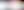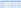## Pratice Numerical Computation Test# Free Practice Numerical Computation Tests

Updated May 2021

## Online Numerical Tests on Job Test Prep

Numerical Computation printable PDF on Psychometric Success:

Numerical computation questions test your ability to use the basic principles of arithmetic like addition, subtraction, multiplication and division.

They may also use mathematical terms and methods such as decimals, percentages, ratios, roots, fractions, powers and exponents.

These questions make no attempt to test your numerical reasoning ability

The method you need to get the correct answer will be obvious and to score well on these questions you will simply need to make quick and accurate calculations.Practice Numerical Computation Test

This sample question paper contains 40 questions and has a suggested time limit of 10 minutes.

The questions are presented in Letter/A4 format for easy printing and self-marking.

If you are very rusty with arithmetic, try re-learning the times tables up to 12 and practice multiplication, division and percentage calculations.

Practice can improve your test scores for all types of aptitude tests, so try as many examples as you can.

We recommend the numerical test packages from JobTestPrep.

These questions are directly applicable to many administrative and clerical jobs but can also appear as a component of graduate and managerial tests.

The speed at which you can answer these questions is the critical measure, as most people could achieve a very high score given unlimited time in which to answer.

You can therefore expect 25-35 questions in 20-30 minutes.